# Discovering Euler's Totient Function

 Part 1 Part 2

Back in the days when I was in the final year of obtaining the Bachelor's degree, we had an amazing professor, Dr. Narsimhan. He had founded the Maths Club. After the regular lectures, all the math professors gathered in a class room & discussed any random interesting math topic. The club was open to anybody who was interested to attend.
It was one of those Math Club lectures where we were solving some problems from IIT Math Olympiad question papers. Dr. Narsimhan had moved on to some other college, by then. As a part of solving one of the questions, we needed to find number of numbers that are coprime to 10,000. Here is an article on coprime numbers, if you are new to the topic.
To explain it in a couple of lines, coprime numbers are relatively prime numbers, two numbers are coprime if their g.c.d is 1.
Eg: Greatest Common Divisor of 15 & 8 is 1. Hence 15 & 8 are coprime. How many numbers less than 15 are coprime to 15? In other words, how many numbers, less than 15 have a gcd of 1 relative to 15?
Let's see :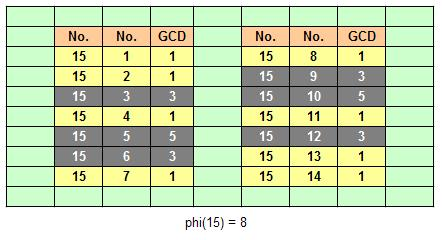From the table above, we find that numbers (yellow background) 1, 2, 4, 7, 8, 11, 13, 14 have gcd of 1 relative to 15. So we say, 1, 2, 4, 7, 8, 11, 13, 14 are all coprime to 15.
How many are they?
There are 8 numbers, in all, that are coprime to 15. Symbolically, we write this as phi(15) = 8 or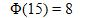Going back to finding the coprime of 10000, we did not have a ready answer then & the question remained un-answered. The question haunted me on my way back home. Not able to resist the temptation to find the answer, I worked out a few examples & stumbled upon a formula to find the number of coprimes for any given number. I had a fair understanding of how far Mathematics had advanced by then, and I felt sure this formula would have been already discovered. Nonetheless, I was excited about the discovery. An year later, I learnt that this formula was first discovered by Euler, and it is called Euler's Totient function. Though Euler had proved this using more sophisticated methods, I happened to work it out using more trivial methods.

Let's go through a couple of examples, before we try our hands at the proof.
Example: Let's say we have to find the number of numbers coprime to 144. Or, in other words, we have to find phi(144).
Let's see how we can do that. We can write factor 144 as 2 x 2 x 2 x 2 x 3 x 3. Or, expressing in terms of powers of primes, 144 = 2^4 x 3^2.
Here, the numbers 2 & 3 are primes. In other words, to express 144 as a product of its prime factors, we only have to use the primes 2 & 3. Now, this is a significant observation, in order to reach our final formula. Next, we write 144 as an array of numbers, having 2 x 3 = 6 rows & 24 columns. (Note: 6 x 24 = 144). The idea is depicted in the table below.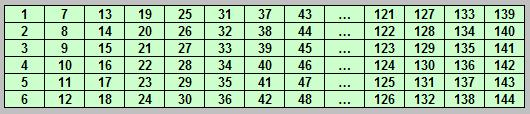The last number in the first column is 6 (which is 2x3).
What are the numbers coprime to 6, in the first column? They are 1 & 5.
Let's move over to the second column.
The last number is 12, which is 6x2.
What are the numbers in the second column, coprime to 12? They are 7 & 11.
Similarly, if we continue the process, we find that the numbers in the first row, viz. 1,7,13, etc... are all coprime to the numbers at the end of the respective columns. Also, the numbers in the 5 th row, 5,11,17,etc... are all coprime to the numbers at the end of the respective columns.
Let's highlight the 2 rows.Now, note that, if a number is coprime to 6, it is also coprime to 144. If any number is coprime to 12, it is also coprime to 144. A number that is coprime to 18, is also coprime to 144.
Which means, any number that is coprime to ANY MULTIPLE of 6, is also coprime to 144.
Knowing this, looking at the table above, all numbers in the highlighted row are coprime to 144.
Now, how many are they? There are 2 in each column. And there are 24 columns in all. Hence there are 24 x 2 = 48 numbers coprime to 144.
That is, phi(144) = 48.
Cool! Following the process described above, we were able to find phi(144).
Now let's try to find phi(10000) using the same process.
First, let's write 10000 in terms of its prime factors : 10000 = 2^4 x 5^4.
The only unique primes needed to express 10000 as a product of primes is 2 and 5.
Next, let's make an array of 2x5=10 rows and 1000 columns, as in picture below.Now, the last number in the first column is 10.
How many numbers in the first column are coprime to 10? That's easy, 4 numbers viz. 1,3,7,9.
The last number in the second column is 20.
How many numbers in the second column are coprime to 20? Again, 4 numbers viz. 11,13,17,19.
Continuing this process, we find that all numbers in the 1st, 3rd, 7th & 9th row are coprime to the last number in that column.
Let's highlight these rows.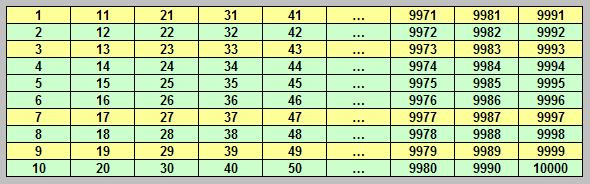Now, all these numbers are also coprime to 10000.
Let's count them. 4 in every column & there are 1000 columns. Hence there are 4000 such numbers, all of which are coprime to 10000.
So there's the answer we were trying to find - phi(10000) = 4000. :-)
That's even cooler. Using this process, we were able to find Phi(10000) under a minute!

So how do these examples lead us to the general formula? Let's work out.
From the first example,
phi(144) = Number of columns * phi (6), which is
= 24 * phi(6)
= (2^3 * 3^1) * phi(2 x 3)
That is,
phi(2^4 * 3^2) = (2^3 * 3^1) * phi(2 x 3)
So, we keep only one power of prime factors within the phi() and take out remaining ones.
Let's see if we come across the same pattern in 2nd example,
phi(10000) = Number of columns * phi (10)
= 1000 x phi(2 x 5)
= (2^3 * 5^3) * phi(2 x 5)
Hence,
phi(2^4 * 5^4) = (2^3 * 5^3) * phi(2 x 5)
Which follows the same pattern as above.

We can thus generalize the formula as
phi (p1^n1 * p2^n2 * p3^n3 ... pk^nk) = [ p1^(n1-1) * p2^(n2-1) * ... * pk^(nk-1) ] * phi (p1 * p2 * p3 * ... * pk)
The formula can be further simplified, as we will see below.

What we saw so far, were examples based on the actual working out of the proof.
I am tempted to post the actual proof, but the proof just follows the same steps as above and we run into numerous symbols that would not be of much interest to a reader who is just seeking some mathematical entertainment. However, I am posting here the generalized theorem & the generalized result.
What follows is the derivation of Euler's Totient function based on the generalized result.

Theorem :
The number of numbers coprime to a number n, whose prime factorization is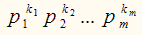denoted by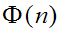is equal toIt can be further shown that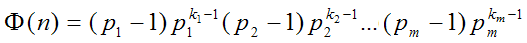Proof:
The first part of the proof follows the same steps as shown in the examples above. Here's the derivation of the second part of the theorem, which leads us to Euler's Totient function.
From above, we have proved that,
phi(n)= p1^(k1-1).p2^(k2-1)...pm^(km-1).phi(p1.p2...pm) ---- (1)
It is known that the function phi() is multiplicative.
That is, if a & b are coprime, then phi(a.b) = phi(a).phi(b) ---- (2)
From (1) & (2), since p1,p2...pm are all primes,
phi(p1.p2...pm) = phi(p1).phi(p2)...phi(pm) ---- (3)
Now, if p is a prime, then ALL numbers less than p are coprime to p.
Which means, phi(p) = p-1
Using the above result in (3), we get
phi(p1.p2...pm) = (p1-1).(p2-1)...(pm-1) ----- (4)
Using (4), equation (1) becomes.
phi(n) = p1^(k1-1).p2^(k2-1)...pm^(km-1).(p1-1).(p2-1)...(pm-1)
Rearranging the terms, we get
phi(n) = (p1-1)p1^(k1-1).(p2-1)p2^(k2-1)...(pm-1)pm^(km-1)
Which is nothing but Euler's Totient function.

Phew! That required some work, didnt it. If you are reading this line, then apparently, you havent dozed off yet.
Thanks! :-) Feel free to write to me & I would be glad to answer any questions you may have. Also, if you are interested in learning more, here is an enlightening discussion about totient function with one of our readers.

 Quick Comment : * Name Email ( Your email id is safe with us! ) * Comments * Type the number of characters in the word "HUMAN"